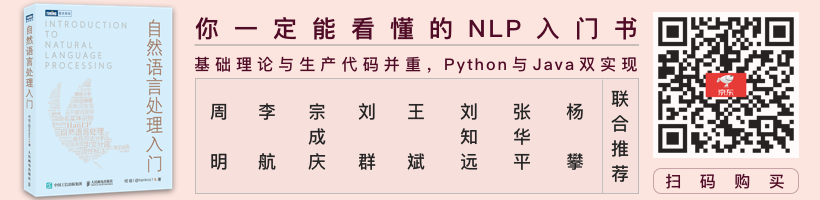谷歌深度学习公开课任务 2: SGD任务 2: 随机梯度下降

安装TensorFlowMac OS X Python 3.5 下的安装方法：

pip3 install --upgrade \$TF_BINARY_URL

测试TensorFlow

python3
Python 3.5.2 (default, Sep 15 2016, 07:38:42)
[GCC 4.2.1 Compatible Apple LLVM 7.3.0 (clang-703.0.31)] on darwin
Type "help", "copyright", "credits" or "license" for more information.
>>> import tensorflow as tf
>>> hello = tf.constant('Hello, TensorFlow!')
>>> sess = tf.Session()
>>> print(sess.run(hello))
b'Hello, TensorFlow!'
>>> a = tf.constant(10)
>>> b = tf.constant(32)
>>> print(sess.run(a + b))
42

TensorFlow入门

graph = tf.Graph()
with graph.as_default():
...

with tf.Session(graph=graph) as session:
...

# With gradient descent training, even this much data is prohibitive.
# Subset the training data for faster turnaround.
train_subset = 10000

graph = tf.Graph()
with graph.as_default():
# Input data.
# Load the training, validation and test data into constants that are
# attached to the graph.
tf_train_dataset = tf.constant(train_dataset[:train_subset, :])
tf_train_labels = tf.constant(train_labels[:train_subset])
tf_valid_dataset = tf.constant(valid_dataset)
tf_test_dataset = tf.constant(test_dataset)

# Variables.
# These are the parameters that we are going to be training. The weight
# matrix will be initialized using random values following a (truncated)
# normal distribution. The biases get initialized to zero.
weights = tf.Variable(
tf.truncated_normal([image_size * image_size, num_labels]))
biases = tf.Variable(tf.zeros([num_labels]))

# Training computation.
# We multiply the inputs with the weight matrix, and add biases. We compute
# the softmax and cross-entropy (it's one operation in TensorFlow, because
# it's very common, and it can be optimized). We take the average of this
# cross-entropy across all training examples: that's our loss.
logits = tf.matmul(tf_train_dataset, weights) + biases
loss = tf.reduce_mean(
tf.nn.softmax_cross_entropy_with_logits(logits, tf_train_labels))

# Optimizer.
# We are going to find the minimum of this loss using gradient descent.

# Predictions for the training, validation, and test data.
# These are not part of training, but merely here so that we can report
# accuracy figures as we train.
train_prediction = tf.nn.softmax(logits)
valid_prediction = tf.nn.softmax(
tf.matmul(tf_valid_dataset, weights) + biases)
test_prediction = tf.nn.softmax(tf.matmul(tf_test_dataset, weights) + biases)

num_steps = 801

def accuracy(predictions, labels):
return (100.0 * np.sum(np.argmax(predictions, 1) == np.argmax(labels, 1))
/ predictions.shape)

with tf.Session(graph=graph) as session:
# This is a one-time operation which ensures the parameters get initialized as
# we described in the graph: random weights for the matrix, zeros for the
# biases.
tf.initialize_all_variables().run()
print('Initialized')
for step in range(num_steps):
# Run the computations. We tell .run() that we want to run the optimizer,
# and get the loss value and the training predictions returned as numpy
# arrays.
_, l, predictions = session.run([optimizer, loss, train_prediction])
if (step % 100 == 0):
print('Loss at step %d: %f' % (step, l))
print('Training accuracy: %.1f%%' % accuracy(
predictions, train_labels[:train_subset, :]))
# Calling .eval() on valid_prediction is basically like calling run(), but
# just to get that one numpy array. Note that it recomputes all its graph
# dependencies.
print('Validation accuracy: %.1f%%' % accuracy(
valid_prediction.eval(), valid_labels))
print('Test accuracy: %.1f%%' % accuracy(test_prediction.eval(), test_labels))

tf.global_variables_initializer().run()

Traceback (most recent call last):
File "udacity-deep-learning/2_fullyconnected.py", line 142, in <module>
tf.global_variables_initializer().run()
AttributeError: module 'tensorflow' has no attribute 'global_variables_initializer'

tf.initialize_all_variables().run()

Loss at step 800: 1.066299
Training accuracy: 79.6%
Validation accuracy: 75.0%
Test accuracy: 83.0%

# With gradient descent training, even this much data is prohibitive.
# Subset the training data for faster turnaround.
train_subset = 10000

with tf.Session(graph=graph) as session:
tf.global_variables_initializer().run()
print("Initialized")
for step in range(num_steps):
# Pick an offset within the training data, which has been randomized.
# Note: we could use better randomization across epochs.
offset = (step * batch_size) % (train_labels.shape - batch_size)
# Generate a minibatch.
batch_data = train_dataset[offset:(offset + batch_size), :]
batch_labels = train_labels[offset:(offset + batch_size), :]
# Prepare a dictionary telling the session where to feed the minibatch.
# The key of the dictionary is the placeholder node of the graph to be fed,
# and the value is the numpy array to feed to it.
feed_dict = {tf_train_dataset : batch_data, tf_train_labels : batch_labels}
_, l, predictions = session.run(
[optimizer, loss, train_prediction], feed_dict=feed_dict)

Minibatch loss at step 3000: 1.182136
Minibatch accuracy: 75.0%
Validation accuracy: 79.0%
Test accuracy: 86.6%

题目1024个隐藏层节点的神经网络。

batch_size = 128
hidden_size = 1024

graph = tf.Graph()
with graph.as_default():
# Input data. For the training data, we use a placeholder that will be fed
# at run time with a training minibatch.
tf_train_dataset = tf.placeholder(tf.float32,
shape=(batch_size, image_size * image_size))
tf_train_labels = tf.placeholder(tf.float32, shape=(batch_size, num_labels))
tf_valid_dataset = tf.constant(valid_dataset)
tf_test_dataset = tf.constant(test_dataset)

# Variables.
W1 = tf.Variable(tf.truncated_normal([image_size * image_size, hidden_size]))
b1 = tf.Variable(tf.zeros([hidden_size]))

W2 = tf.Variable(tf.truncated_normal([hidden_size, num_labels]))
b2 = tf.Variable(tf.zeros([num_labels]))

# Training computation.
y1 = tf.nn.relu(tf.matmul(tf_train_dataset, W1) + b1)
logits = tf.matmul(y1, W2) + b2

loss = tf.reduce_mean(
tf.nn.softmax_cross_entropy_with_logits(logits, tf_train_labels))

# Optimizer.

# Predictions for the training, validation, and test data.
train_prediction = tf.nn.softmax(logits)

y1_valid = tf.nn.relu(tf.matmul(tf_valid_dataset, W1) + b1)
valid_logits = tf.matmul(y1_valid, W2) + b2
valid_prediction = tf.nn.softmax(valid_logits)

y1_test = tf.nn.relu(tf.matmul(tf_test_dataset, W1) + b1)
test_logits = tf.matmul(y1_test, W2) + b2
test_prediction = tf.nn.softmax(test_logits)

# Let's run it:
num_steps = 3001

with tf.Session(graph=graph) as session:
tf.initialize_all_variables().run()
print("Initialized")
for step in range(num_steps):
# Pick an offset within the training data, which has been randomized.
# Note: we could use better randomization across epochs.
offset = (step * batch_size) % (train_labels.shape - batch_size)
# Generate a minibatch.
batch_data = train_dataset[offset:(offset + batch_size), :]
batch_labels = train_labels[offset:(offset + batch_size), :]
# Prepare a dictionary telling the session where to feed the minibatch.
# The key of the dictionary is the placeholder node of the graph to be fed,
# and the value is the numpy array to feed to it.
feed_dict = {tf_train_dataset: batch_data, tf_train_labels: batch_labels}
_, l, predictions = session.run(
[optimizer, loss, train_prediction], feed_dict=feed_dict)
if (step % 500 == 0):
print("Minibatch loss at step %d: %f" % (step, l))
print("Minibatch accuracy: %.1f%%" % accuracy(predictions, batch_labels))
print("Validation accuracy: %.1f%%" % accuracy(
valid_prediction.eval(), valid_labels))
print("Test accuracy: %.1f%%" % accuracy(test_prediction.eval(), test_labels))weights = tf.Variable(tf.truncated_normal([image_size * image_size, num_labels]))
biases = tf.Variable(tf.zeros([num_labels]))
logits = tf.matmul(tf_train_dataset, weights) + biases
loss = tf.reduce_mean(tf.nn.softmax_cross_entropy_with_logits(logits, tf_train_labels))

# Variables.
W1 = tf.Variable(tf.truncated_normal([image_size * image_size, hidden_size]))
b1 = tf.Variable(tf.zeros([hidden_size]))

W2 = tf.Variable(tf.truncated_normal([hidden_size, num_labels]))
b2 = tf.Variable(tf.zeros([num_labels]))

# Training computation.
y1 = tf.nn.relu(tf.matmul(tf_train_dataset, W1) + b1)
logits = tf.matmul(y1, W2) + b2

loss = tf.reduce_mean(tf.nn.softmax_cross_entropy_with_logits(logits, tf_train_labels))

Minibatch loss at step 3000: 1.465061
Minibatch accuracy: 83.6%
Validation accuracy: 81.4%
Test accuracy: 88.6%

1. #1

机器要跟得上